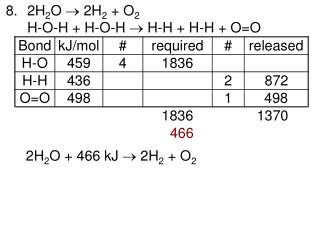DownloadDownload Presentation2H 2 O  2H 2 + O 2 H-O-H + H-O-H  H-H + H-H + O=O

# 2H 2 O  2H 2 + O 2 H-O-H + H-O-H  H-H + H-H + O=O

Download Presentation## 2H 2 O  2H 2 + O 2 H-O-H + H-O-H  H-H + H-H + O=O

- - - - - - - - - - - - - - - - - - - - - - - - - - - E N D - - - - - - - - - - - - - - - - - - - - - - - - - - -
##### Presentation Transcript

1. Bond kJ/mol # required # released H-O H-H O=O 459 4 1836 • 2H2O  2H2 + O2 H-O-H + H-O-H  H-H + H-H + O=O 436 2 872 498 1 498 1836 1370 466 2H2O + 466 kJ  2H2 + O2

2. Bond kJ/mol # required # released H-H Cl-Cl H-Cl • Forming a bond releases energy • Breaking a bond requires energy • The energy required to break a chemical bond: the energy released when a bond is formed • Endothermic • Exothermic • a) H-F (568 kJ/mol) b) O-O (145 kJ/mol) 436 1 436 243 1 243 432 2 864 679 864 185 H2 + Cl2 2HCl + 185 kJ

3. Bond kJ/mol # required # released C-C C-H C=O H-O O=O 348 24 8352 • a) O=O, H-O-H, O=C=O b) C25H52 + 38O2 25CO2 + 26H2O c) 413 52 21476 799 50 39950 459 52 23868 498 38 18924 48752 63818 15066 d) theoretical: 15000 kJ, lab: 7500 kJ The lab value is about 50% of what it should have been because the calorimeter was very inefficient – lots of heat energy was lost.

4. Bond kJ/mol # required # released C-C C-H C-O H-O O=O C=O 348 1 348 413 5 2065 • C2H5OH + 3O2 2CO2 + 3H2O 360 1 360 459 1 459 6 2754 498 3 1494 799 4 3196 4726 5950 1224 C2H5OH + 3O2 2CO2 + 3H2O + 1224 kJ Thus,molar heat of combustion,Hc=1224 kJ/mol h = 1224 kJ/mol x 1 mol/46.08 g = 26.6 kJ/g For more lessons, visit www.chalkbored.com